# 50W Current-Mode Forward Converter Design with the MAX8540

### 要約

This application note details the design of a 50-watt, isolated, forward converter, using the MAX8540 synchronizable, high-frequency, current-mode PWM controller. Design procedures for both the power stage and controller are presented, along with actual performance measurements.

The converter delivers 20A of Load current at an output voltage of 2.5V. It employs synchronous rectifiers for secondary rectification. The input voltage range for the converter is 36-75VDC.

This design is available as an evaluation board. The evaluation board demonstrates how easy it is to implement the features network and telecom applications require. The design methods can easily be adapted to the design of high performance, full featured off-line power supplies.

Key features of the application are:

• 300kHz switching frequency
• Programmable input UV/OV Protection
• Programmable hiccup current limit or latch mode overcurrent protection
• Programmable maximum duty cycle clamp with feedforward
• Programmable slope compensation with single resistor
• Synchronization to external clock
• Active low-enable feature for easy turn on/off of converter
• Internal leading edge blanking on the current sense pin
• Output overvoltage protection
• Space-saving 16 pin QSOP

### Description of Application Circuit Operation

Figure 1 shows the circuit diagram of a 2.5V, 20 A isolated forward converter that uses the MAX8540 current mode controller (U1). At startup, the total capacitance at the Vcc pin is charged through MOSFET Q7 and the parallel combination of resistors R30 and R22 from the dc input voltage VIN. When Vcc exceeds the undervoltage lockout threshold of the MAX8540, it goes through the soft-start mode and pulses of gradually increasing duty cycle are applied to the gate drive IC, U8. The MOSFET Q1 therefore starts to switch the input DC voltage across the power transformer T1, used to provide isolation and to step down the input DC voltage to the required level. (Selection of power transformer turns ratio is dealt with in the following section.) Since the energy for driving Q1 comes from the capacitance at Vcc, the Vcc voltage falls. The hysteresis of the MAX8540 undervoltage lockout feature allows this to happen. Pulses on the bias winding of transformer T1 are rectified by D1, regulated, and applied to the Vcc pin. The rectified and regulated bias circuit voltage builds up and prevents the Vcc pin from falling below the undervoltage lockout threshold. The primary side control continues to operate from the bias winding. The MAX8515 (U2) is configured to sense the Vcc pin and turn off Q7 at a voltage slightly higher than the worst-case startup voltage for U1. This avoids unnecessary power dissipation in Q7, R30, and R22.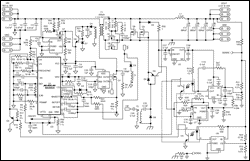More Detailed Image

Figure 1. Circuit diagram of 2.5 V, 20 Amp, Isolated Current mode Forward Converter using the MAX8540.

For power transformer T1, the volt-seconds applied to the primary winding during the "ON" time of Q1 should be balanced by the volt-seconds applied during the "OFF" time, in order to maintain the operating point for the flux in the core. This is achieved by employing D2 and a "demagnetizing winding" whose number of turns equally the primary turns, and is connected with polarity as shown in the schematic. When Q1 turns off, D2 conducts the magnetizing current and the polarity of the applied voltage to the demagnetizing winding causes the magnetizing current to decay to zero. Since the magnitude of voltage applied during the ON and OFF times is identical, the ON time equals the time taken for the magnetizing current to decay to zero and "reset" the core. This limits the duty cycle to a maximum value of 50%, beyond which proper reset of the core will not occur, leading to saturation of the core.

The high frequency switching waveform appearing across the primary of T1 is stepped down by T1 and rectified by synchronous rectifiers Q2, Q8, Q3 and Q9. The forward synchronous rectifiers Q2, Q8 are self-driven from the secondary winding of T1. The freewheeling rectifiers Q3, Q9 are driven with an inverted, suitably delayed version of the gate drive pulses to Q1, using transformer T2. Q5 provides for fast turn off of Q3, Q9. The rectified pulse train is applied to the output L-C filter L1, C11, C12, C13 and C26. The output voltage of the L-C filter is the Average value of the rectified pulse train. For a fixed frequency switching scheme as implemented in the MAX8540, the output voltage is proportional to the "ON" time of the rectified pulse train. The feedback circuit consisting of U4 (LMX321), U5 (LP2980), and U7 (MAX8515) perform the function of regulating the output voltage for load and dc input voltage variations. U4 is a low dropout linear regulator that provides a fixed bias for the secondary side feedback circuit. The output voltage is sensed by resistor divider R12, R11 and is applied to the inverting input of op-amp U4. U7 provides the reference voltage to the non-inverting pin of U4. At start-up the reference is applied through an R-C delay (R36, C29) so as to produce a smooth output voltage start-up waveform. The error between the reference and the output voltage drives the (pins 1, 2) LED portion of optocoupler U3, which couples the error signal across the isolation boundary. The phototransistor (pins 8, 7 of U3) produces a current that depends on the current transfer ratio of U3 and adjusts the voltage at the OPTO pin of U1 to program the peak primary current and therefore the output current required to produce the desired output voltage. The primary current is sensed by means of resistor R8. U6 (MAX8515) provides the output overvoltage protection feature for the converter. When the output voltage exceeds 2.87 volts, the OUT pin of U6 goes low and drives the (pins 3,4) LED portion of U3 to turn on Q4 and shutdown the converter. This initiates a fresh start-up cycle for the converter.

### Transformer Design

Once the required core size for the given power output, switching frequency, flux density, and temperature rise have been established, the primary to secondary turns ratio is estimated. In the typical application circuit, the maximum allowed duty ratio is 50%, due to the transformer core reset scheme employed. Therefore the primary to secondary turns-ratio ns/np should be based on the lowest operational input voltage, as follows,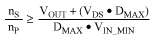where VOUT is the output voltage, VDS is the voltage drop across the synchronous rectifier, DMAX is the maximum allowable duty ratio (use 0.45 for some safe margin), and VIN MIN is the minimum operational input voltage. The design for the actual number of primary turns for low voltage, high current "bricks" for the telecom input voltage range is done by assuming 1 turn for the secondary. This approach is especially true of the "core-on-board" transformers designed for these applications. The turns-ratio from primary winding to primary bias winding is given by,,

Where VD is the voltage drop across the bias winding diode. At the minimum operational input voltage the bias voltage should be at least 9V to power up the MAX8540 and it is a good trade-off between the driving voltage and efficiency.

The reset winding should have the same number of turns of the primary winding, however small gauge wire can be used because the rms current through the reset winding is very small.

To construct the transformer, one needs to know the rms currents for both primary and secondary windings. These are given as follows.where IOUT is the maximum output current.

Once the above parameters are known, the transformer can be designed. Tightly wound the primary and the reset windings together help to minimize the switching loss due to the leakage inductance at each time when the transformer is reset. Interleaving primary and secondary windings help to increase the coupling and reduce the leakage inductance. However, it may increase the cost if the transformer needs to meet safety requirement. In the typical application circuit however, a standard off-the-shelf transformer is used. The primary to secondary turns ratio for the selected transformer is 0.188.

### Output Inductor Selection

There are several parameters that must be examined when determining an optimum inductor value. Input voltage, output voltage, load current, switching frequency and LIR. LIR is the ratio of inductor current ripple to DC load current. A higher LIR value allows for a smaller inductor, but results in higher losses and higher output ripple current. A good compromise between size, efficiency and cost is a LIR of 30%. Once all of the parameters are chosen, the inductor value is determined as follows:Where VSEC is the voltage on the secondary side of the transformer at which the maximum ripple voltage is specified and fS is the switching frequency. Choose a standard value close to the calculated value. For the application circuit, plugging in the values for the above equation, and selecting the nearest standard inductor results in a value of 2.2µH. Lower inductor values minimize size and cost, but they also increase the output ripple and reduce the efficiency due to higher peak currents. On the other hand, higher inductor values increase efficiency, but eventually resistive losses due to extra turns of wire will exceed the benefit gained from lower AC current levels. For any area-restricted applications, find a low-core-loss inductor having the lowest possible DC resistance. Ferrite cores are often the best choice. The chosen inductor's saturation current rating must exceed the expected peak inductor current (IPEAK). Consult the inductor manufacturer for saturation current ratings. Determine IPEAK as:where VSEC is the maximum secondary side voltage.

### Output Capacitor Selection

As in any high-frequency power supply, the output filter capacitors must meet very low ESR and ESL requirements. At the 300kHz frequency, the most favorable technologies are ceramic capacitors and polymer capacitors (POSCAPs). The key selection parameters for the output capacitor are capacitance, ESR, ESL and the voltage rating requirements. It may be noted that capacitance, ESR and voltage rating are also temperature dependent. These parameters affect the overall stability, output ripple voltage and transient response of the DC-DC converter. The output ripple occurs due to variations in the charge stored in the output capacitor, the voltage drop due to the capacitor's ESR, and the voltage drop due to the capacitor's ESL. Calculate the output voltage ripple due to the output capacitance, ESR and ESL as: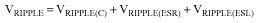Where the output ripple due to output capacitance, ESR, and ESL are: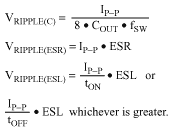And IP-P the peak-to-peak inductor current is:The peak values estimated by the above equations for the three components of ripple voltage are not in phase, and therefore cannot be added algebraically. Usually, one of the ripple components dominates the others and can be used for initial capacitor selection. As a rule, a smaller ripple current results in less output voltage ripple. Since the inductor ripple current is a factor of the inductor value, the output voltage ripple decreases with larger inductance. Load transient response depends on the selected output capacitors. During a load transient, the output instantly changes by ESR x ILOAD. Before the controller can respond, the output deviates further, depending on the inductor and output capacitor values. After a short time, the controller responds by regulating the output voltage back to its nominal state. The controller response time depends on the closed-loop bandwidth. A higher bandwidth yields a faster response time, thus preventing the output from deviating further from its regulating value. For the application circuit, 3 x 680µF, POSCAPs are used, each with an ESR of .035 Ω.

### Input Capacitor Selection

The input capacitor (CIN) reduces the current peaks drawn from the battery or input power source. The impedance of the input capacitor at the switching frequency should be less than that of the input source so that high-frequency switching currents are supplied by the input capacitor rather than from the source. The input capacitor must meet the ripple current requirement (IRMS) imposed by the switching currents. Non-tantalum chemistries (ceramic, aluminum, or organic) are preferred due to their resistance to power-up surge currents. IRMS is calculated as follows:,

where N is the primary to secondary turns ratio. For the forward converter, VIN is the minimum input voltage for designs where the maximum duty ratio is less than 0.5, and the value of input voltage at which the duty ratio equals 0.5 for designs with maximum duty ratio greater than 0.5. Choose input capacitors that have a higher ripple current rating than the calculated value. For the application circuit, 3 x 0.47uF/100V ceramic caps are used.

### Primary MOSFET Selection

The MAX8540 typically drives an n-channel MOSFET power switch. The maximum drain voltage, maximum RDS(ON) and total gate switching charge are the parameters involved in choosing the FET. The maximum gate switching charge is an important factor defining the power consumption, since the product of the switching frequency and the total gate charge is the current consumption of the MAX8540 controller. RDS(ON) is the parameter that determines the total conduction power losses in the switch and the choice depends on the expected efficiency and the cooling and mounting method. The maximum drain voltage requirements can be different depending on the transformer reset scheme used. For the forward converter show in the application circuit, a simple demagnetizing winding based reset scheme is used, wherein the maximum voltage stress on the MOSFET switch is 2 times the highest input voltage. Allowing for leakage inductance spike, a 200V MOSFET should be used. The MOSFET should also handle the RMS current associated with the forward topology. The current through the MOSFET is determined as: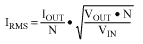A MOSFET with the lowest total gate charge and the lowest RDS(ON) for the maximum drain voltage expected (plus some safety factor) is the best choice. The choice of package depends on the application, the total power and the cooling methods available. For the Application circuit, based on the above considerations, IRF640 MOSFET, 200 Volts, 18 Amps, RDS(ON)=0.18Ω is chosen.

### Secondary Synchronous Rectifier Selection

The typical Application circuit uses synchronous rectifier for both the forward and the freewheeling rectifiers on the secondary side. The forward synchronous rectifier is self-driven from the secondary winding, and freewheeling rectifier is driven from a gate drive transformer with signals generated by the controller IC. The voltage rating for the synchronous rectifiers is equal to the maximum secondary voltage plus a margin for spike due to leakage inductance. Switching losses in this topology are not an issue due to the lower Drain-Source voltages. For synchronous rectifiers, the power dissipation is mainly due to conduction losses. The power dissipation is calculated as: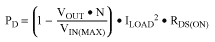,

for the freewheeling rectifier, and,

for the forward synchronous rectifier. Choose MOSFETs with RDS(ON) such that an acceptable junction temperature is achieved for the estimated power dissipation. Note the synchronous rectifiers' maximum junction temperature depends on the thermal resistance that will be realistically achieved with the device packaging, layout and cooling methods used. In the application circuit, 2 x IRF7832, 30V, 20A, RDS(ON)=4mΩ @ Vgs=10V MOSFETs are used for both forward and freewheeling synchronous rectifiers.

### OV Threshold

The MAX8540 includes an over-voltage protection feature that turns off the external MOSFET when the input voltage exceeds the user-set threshold. Connect a resistor divider from the system input to GND with OV connected to the center to set the over-voltage protection trip point. The threshold voltage for OV is 3.021V (typ).where VOV is the OV threshold, VIN(MAX) is the over-voltage trip-point, R1 is the resistor from the system input to OV and R2 is the resistor from OV to GND.

### UV Threshold

The MAX8540 also includes an under-voltage sensing input. The IC holds the external MOSFET low until UV reaches its threshold (1.25V typ). Once the threshold has been reached, the circuit enters soft-start and brings the output into regulation. Connect a resistor divider from the system input to GND with UV at the center to set the under-voltage protection trip point.where VUV is the UV threshold, VIN(MIN) is the under-voltage trip-point, R1 is the resistor from the system input to UV and R2 is the resistor from UV to GND.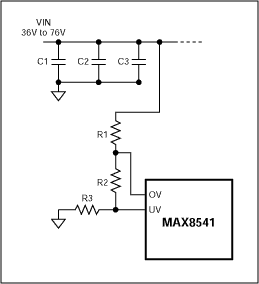An alternate method used in the application circuits for setting the over-voltage and under-voltage trip points is demonstrated in Figure 2. Use 36.5kΩ for the bottom resistor (R3). R2 and R1 are calculated as follows:Where VIN(MIN) is the under-voltage trip point, VIN(MAX) is the over-voltage trip point, VUV is the UV threshold (1.25V typ) and VOV is the OV threshold (3.021V typ). R1 should consist of two equal value resistors in series to protect against single point failure.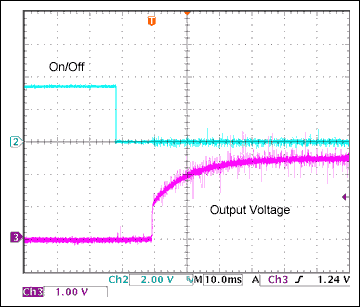Figure 2. Output voltage at startup using the ON/OFF feature of 8540.

Table 1. Typical specifications of UV/OV and the actual on/off hysteresis of input voltage.

 UV voltage specs VIN Off Window (LTP) VIN On Window (UTP) MIN 1.083 29.76V 31.00V32.24V TYP 1.128 MAX 1.173 MIN 1.200 32.97V34.34V35.70V TYP 1.250 MAX 1.300 OV voltage specs VIN Off Window (UTP) VIN On Window (LTP) MIN 2.901 79.70V83.00V86.32V TYP 3.021 MAX 3.142 MIN 2.778 76.32V79.50V82.68V TYP 2.894 MAX 3.010

Assume that 1.250V at UV/OV is scaled to 34.34V for 48V bus and 17.17V for 24V bus.

For achieving the input OVP and input UVP trip points as shown in Table 1, the above equations results in R1=965k, R2=402Ω, and R3=36.5k.

### Switching Frequency and Synchronization

The MAX8540 oscillator operates in two modes: stand-alone or synchronized (sync). A single input, FREQ/SYNC, doubles as the attachment point for the frequency programming resistor and as the synchronization input. The mode recognition is automatic, based on the signal applied to FREQ/SYNC. In stand-alone mode, an external resistor connected from FREQ/SYNC to GND sets the operating frequency. A 1.25V source is internally applied to FREQ/SYNC and the oscillator frequency is proportional to the current out of FREQ/SYNC through the programming resistor. The operating frequency is determined as:The MAX8540 also synchronizes with an external oscillator. Drive FREQ/SYNC with a square wave with a positive pulse width of at least 200ns and a minimum pulse amplitude of 3V plus the VF of the external diode. The maximum duty cycle of the external signal allowed is 55%. The MAX8540 synchronizes to frequencies between 200kHz and 1MHz, however, the signal must be within ±30% of the frequency set by the external resistor at FREQ/SYNC. Frequency set resistor R4 from the above is calculated to be 32.4k. An R-C filter as specified in the application circuit should be connected across R4 for nose filtering.

### Maximum Duty Cycle

Set the maximum duty cycle at the minimum system input voltage (VIN(MIN)) connecting a resistor from MAXDTY to GND. The maximum duty cycle is inversely proportional to the voltage at UV. As the voltage on UV increases the duty cycle decreases. The maximum duty cycle is internally limited to 80% at all switching frequencies. The MAXDTY resistor is determined as:The range of valid resistor values for RMAXDTY is from 24.3kΩ to 130kΩ. For the application circuit, a value of 80.6k is chosen to limit the duty ratio to within 50%.

### N-Channel MOSFET Driver

The DRV output drives an N-channel MOSFET in low power applications. In high power applications, the gate driver internal to the MAX8540 may not be capable of driving the external MOSFET efficiently and an external gate driver may be required. In this situation, connect DRV to the input of the external gate driver.

### Slope Compensation

The MAX8540 is a current-mode device and requires slope compensation for proper operation. To provide slope compensation, connect a resistor from SCOMP to GND (RSCOMP). The value of RSCOMP is determined as following:

For applications using a synchronous rectifier in the output, set the slope compensation equal to the negative slope of the output inductor. RSCOMP is equal to:Where dVRAMP/dt = 2.5V × fS, RSUM=25kΩ, N is the turns ratio np/ns of the primary to secondary, L1 is the output inductance, VOUT is the output voltage, fS is switching frequency, and RCS is the current-sense resistance. For the application circuit, the above equations yield a value of RSCOMP=30.9k. This amount of slope compensation may not be required for converters where the Duty Ratio is less than 0.5, and therefore sub-harmonic oscillations are not a problem. It is to be noted that the more the slope compensation added, the converter tends to behave like a voltage mode converter. Therefore the required amount of slope compensation should be added. For converters where Duty cycle is less than 0.5, adding a small amount of slope to the current signal improves operation at low load currents.

For applications where a diode is used in the output instead of the synchronous rectifier, the slope compensation resistor is then equal to:where VF is the diode voltage drop.

### Soft-Start

The soft-start feature allows converters built using the MAX8540 to apply power to the load in a controllable soft ramp, thus reducing start-up surges and stresses. It also determines power-up sequencing when several converters are used. Upon power turn-on, SS acts as a current sink to discharge any capacitance connected to it. Once the voltage at VCC has exceeded its lockout value, SS then charges the external capacitor (CSS) allowing the converter output voltage to ramp up. Full output voltage is reached in approximately 440ms/µF. Since the application circuit has a secondary soft start which is used to control the output voltage at start-up, the SS delay is set to be minimal. A delay of 660uS is set using a 1500pf capacitor.

### Current-Limit

Two types of current limit schemes can be implemented with the MAX8540. They are the "Hiccup mode" and "Latch" mode. The CS signal provides feedback on the current ramp through the main external MOSFET. The voltage on CS is monitored by the MAX8540. The cycle-by-cycle current-limit feature abbreviates the on-time of the external MOSFET in the event that the voltage at CS is greater than the threshold voltage set by ILIM. Set the current-limit threshold using a resistor divider from REF to GND with ILIM connected to the center. The current-limit threshold is determined as: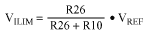Where VREF is the 5V reference and R26 and R10 are the external resistors. Use 10kΩ for R16 and vary R26 to change the threshold. For the Application circuit, R26 was adjusted to 205k to set the current limit at 125% of the full load current. To select Hiccup mode, connect capacitors to SKTON and SKTOFF to program the hiccup mode on- and off-time. When a cycle-by-cycle event is detected, the IC charges the capacitor at SKTON. The capacitor continues to charge as long as the CS voltage is greater than the ILIM threshold voltage. Once the voltage on SKTON reaches its threshold voltage, the MAX8540 begins skipping switching cycles for a time determined by the capacitance connect to SKTOFF. Once this time period has elapsed, the IC begins to switch for the time period set by the capacitance connected to SKTON. This process continues until the output short or overload condition is removed.

To select latched mode, connect SKTOFF to REF. In this mode, if the hard short or overload exceeds the time period set by the capacitance at SKTON, the output is latched off. To unlatch the output, toggle active-low EN or cycle the input power to VCC.

The hiccup mode is chosen for the Application circuit. See the SKTON and SKTOFF section below for details on setting the hiccup mode periods.

### SKTON and SKTOFF

The capacitance, CSKTON, determine the time period allowed before the short-circuit current-limit initiates. Once the CS voltage exceeds the ILIM threshold, the capacitor at SKTON begins to charge. The capacitor continues to charge until the SKTON threshold voltage is reached or the over-current event is removed. This feature allows for the higher currents required during start-up to bring the IC online. Set CSKTON in order to allow sufficient time for start-up. The required capacitance at SKTON is determined as:

CSKTON=tON/10³

Where tON is in ms and CSKTON is in µF. The allowable range for CSKTON is 100pF to 0.01µF

The capacitance at SKTOFF determines the time period that the external MOSFET is turned off during an over-current event. Once the SKTON time period is exceeded, the SKTOFF capacitor charges. Once VSKTOFF reaches its threshold, the IC begins to switch again. CSKTOFF is determined as:

CSKTOFF=tOFF/10³

Where tOFF is in ms and CSKTOFF is in µF. The allowable range for CSKTOFF is 1000pF to 1µF. For the Application circuit, CSKTON=0.0047uF and CSKTOFF=.068uF are used.

Pull VSKTOFF to VREF through a 10kΩ pull-up resistor to enable the latch-off feature. In this mode, once the SKTON time has elapsed, the IC is latched off. The circuit will remain off until active-low EN is toggled, or the input power is toggled.

### Compensation

Since current mode control is employed using the MAX8540 current mode controller, the power stage of the forward converter exhibits a single output pole due to the output capacitor and load combination, along with a zero due to the ESR of the output capacitor. The goal of the compensator design is to achieve a single slope of -20 db/ decade with a phase margin greater than 45 degrees at the crossover frequency. To achieve a good dc regulation, a high low-frequency gain is another requirement for the compensator. To achieve the above requirements, the compensator should have one zero, one pole and an integrator. The type 2 scheme readily achieves this. For the Forward converter in the Application circuit, the open loop gain under current mode control is given by the expression,,

where GOPTO is the optocoupler gain, RL the load resistance, C14, C15, C24, R27, R6 and R14 and R11 are the reference designators are used in the MAX8540 Typical Application Circuits. COUT is the total output capacitance and RESR is the output capacitors' ESR. RCS is the current sense resistor designated as R8 in the Application circuit.

The frequency that the output pole and zero occur at is determined as: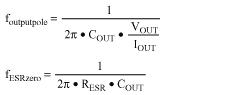From the above equations, the foutputpole and foutputzero are determined as 624Hz and 6.9kHz respectively.

Calculate the compensation components using the following method:

First, determine the desired bandwidth (fBW) of the system. The bandwidth (crossover frequency) will determine the speed that the MAX8540 will respond to changes in the output caused by load transients. A bandwidth of 5kHz is chosen for the Application circuit. Unity gain is desired at fBW. Therefore, T(s) at fBW must be equal to 1. Choose C14=0.1µF, s=2p*fBW and set the loop gain to unity in equation for loop gain T(s) to determine R11. For a single active pole at the crossover frequency,where nP is the number of primary turns, nS is the number of secondary turns, and GOPTO is the gain of the optoisolator used. The value for R11 is obtained as 31.8k from the above equation.

Place the R27 zero to compensate for the pole due to load resistance and output capacitor. The location of the zero is selected to be 3 times higher than the pole location. Exact cancellation of the pole results in a large time constant R27.C14 since the zero has to be placed at a lower frequency. This results in sluggish response during large load transients when the controller saturates. R27 is found to be 910 Ω using the following equation: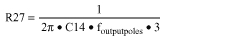The pole in the controller due to C15 and R27 is used to cancel the ESR zero. A value of C15=.022µF is obtained from the following equation:The above method though simple gives compensator values that serve as a good starting point only. During actual testing with a network analyzer, the crossover frequency was found to be lower than desired. This error results from the equation for R11 which assumes exact cancellation of the converter poles and zeros by those of the controller. Therefore R11 was reduced to 15K in order increase gain and achieve the desired crossover frequency. Also optocoupler gain and Phase shift is not considered in this simple design method. The optocoupler exhibits significant phase shift around the crossover and therefore reduces the phase margin. The pole used to compensate the ESR zero in the controller was actually removed in order to achieve the required phase margin of 45 degrees. The final values for the compensator are R11=15k, R27= 910, C14=0.1µF, C15=not used.

### Output Overvoltage Protection

Output overvoltage protection is implemented by sensing the overvoltage condition using the MAX8515 (U6) as a comparator, coupling the fault signal through an optocoupler (U3), and pulling down the UV input of the MAX8540. The MAX8540 turns off the drive pulses and goes through a fresh startup cycle repeatedly till the overvoltage condition is removed.

### Layout Guidelines

All connections carrying pulsed currents must be very short, be as wide as possible and have a ground lane behind them whenever possible. The inductance of these connections must be kept to an absolute minimum due to the high di/dt of the currents in high frequency switching power converters. In the development of prototyping process, multipurpose boards, wire wrap and similar constructive practices are not suitable for these types of circuits; attempts to use them will fail. Instead, used milled PC boards with a ground plane or equivalent techniques. Current loops must be analyzed in any layout proposed and the internal area kept to a minimum to reduce radiated EMI. The use of automatic routers is discouraged for PC board layout generation in the board area where the high-frequency switching converters are located. Designers should carefully review the layout. In particular, pay attention to the ground connections. Ground planes must be kept as intact as possible. The ground planes for the power section of the converter should be kept separate from the logic ground planes except for a connection at the least noisy section of the power ground plane. The power-line filter capacitor and the ground return of the power switch or current sensing resistor must be close together. All ground connections must resemble a star system as much as practical. Thermal management is another important issue to be considered in the design of converters such as that described above. The temperature rise of the components is a strong function of the cooling methods and packaging techniques used. Forced cooling is definitely required for the Application circuit to deliver full power reliably.

### Measurements on the MAX8540 EVKIT

Some important measurements taken on the MAX8540 evaluation kit are presented here. They are:
1. Converter Efficiency (Figure 3)
2. Transient Response (Figure 4), and
3. Output voltage at startup (Figure 2) employing the ON/OFF feature of the MAX8540.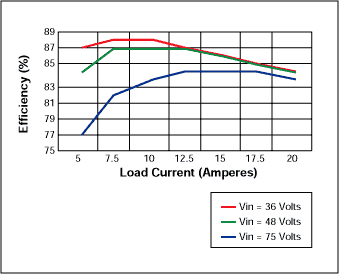Figure 3. Converter efficiency vs load current.Figure 4. Output voltage deviation for step load increase and decrease. Load current slew rate is approximately 0.1 /µS.

### Summary

The design of a 2.5 Volt, 20A converter using the MAX8540 current mode controller has been discussed, and a typical application circuit along with the Bill of materials for the same has been presented. Features particularly desirable for the network and telecom industry incorporated in the MAX8540 have been demonstrated in the Application circuit.

Bill of Material:

 Components Functions Part Number C1, C2, C3 Input filtering capacitor 0.47uF 100V X7R cer cap (1812),TDK, C4532X7R2A474M C4 VCC filtering capacitor 10uF/16V X5R cer cap (1210),Taiyo Yuden EMK325BJ106MN C5 Soft start capacitor 1500pF/50V X7R cer, (0603), Murata,GRM188R71H152KA01B C6 Decoupling for U2 0.1uF/25V, cer, X7R, (0603), GRM188R71E104KA01B C7 VCC bypass capacitor 0.1uF/50V X7R cer cap (0805), Taiyo Yuden UMK212BJ104KG C8 Bias filtering capacitor 1uF/25V, X7R, Taiyo Yuden, TMK316BJ105ML C9 Snubber capacitor 100pF/630V, Murata, GHM1030R101K630 C10 Filter for MAXDTY pin 2200pF/50V X7R cer, (0603), Murata, GRM188R71H222KA01B C11, C12, C13 Output filtering capacitor 680uF/4V/35mΩ, 4TPB680M, POSCAP, Sanyo C14 Loop compensation capacitor 0.1uF/25V, cer, X7R, (0603), GRM188R71E104KA01B C15 Loop compensation capacitor Not used C16 Decoupling capacitor 0.1uF/25V, cer, X7R, (0603), GRM188R71E104KA01B C17 REF bypass capacitor 1uF/10V, Taiyo Yuden, LMK107BJ105MA C18 Time delay capacitor 120pF/50V , COG, ceramic, (0603), GRM1885C1H121JA01B C19 Time delay capacitor 220pF/50V, COG, ceramic, (0603), GRM1885C1H221JA01B C20 Gate drive Xfmr Cap 0.1uF/50V X7R cer cap (0805), Taiyo Yuden UMK212BJ104KG C21 Snubber capacitor 1500pF/50V, X7R, cer, (0603), GRM188R71H152KA01B C22 ON time capacitor at hiccup mode 4.7nF/50V, X7R ceramic, (0603), GRM188R71H472KA01B C23 OFF time capacitor at hicuup mode 0.068uF/25V, cer, (0603), GRM188R71E683KA01B C24 Compensator cap Not used C25 Gate drive Xfmr Cap 0.1µF/ X7R ceramic (0805), Taiyo Yuden UMK212BJ104KG C26 Output filter Capacitor open C27 Bypass Cap for sec bias 0.1uF/25V, X7R, cer, (0603), GRM188R71E104KA01B C28 Bypass Cap for sec bias 1uF/10V, Taiyo Yuden, LMK107BJ105MA C29 Delay cap for sec Ref 1uF/10V, Taiyo Yuden, LMK107BJ105MA C30 Stabilsing cap for U7 1uF/10V, Taiyo Yuden, LMK107BJ105MA C31 capacitor 150pF/50V, X7R ceramic, (0603), GRM1885C1H151JA01B C32 capacitor Open (0603) C33 Decoupling Cap for Driver 1uF/16V, X7R, (0805), TDK, C2012X7R1C105M C34 capacitor 2200pF/50V X7R cer, (0603), Murata, GRM188R71H222KA01B C35 Filter for current lim Ref 120pF/50V, COG, ceramic, (0603), GRM1885C1H101JA01B C36 Filter for feedback signal Open (0603) C37 Decoupling capacitor 0.1uF/25V, cer, X7R, (0603), GRM188R71E104KA01B D1 Rectifying diode 80V/100mA Schottky diode, Panasonic MA111CT, Digikey MA111CT-ND, (S-Mini) D2 Ultrafast rectifier diode 200V/1A, On semiconductor MURA120T3, SMA D3 Zener diode BZX399-1V8, 1.8 volt zener, Phillips semiconductor, (SOD-323) D4 Diode IN4148WS, General Semiconductor, (SOD-323) D5 Diode IN4148WS, General Semiconductor, (SOD-323) D6 Diode IN4148WS, General Semiconductor, (SOD-323) D7 Zener diode 12V Zener diode, Panasonic MA3120CT D8 Diode IN4148WS, General Semiconductor, (SOD-323) D9 Diode IN4148WS, General Semiconductor, (SOD-323) U1 PWM Control IC MAX8540, current mode, 16 PIN QSOP, MAXIM U2 OVP comparator MAX8515, SC-70, MAXIM U3 Dual Opto-coupler Dual 70V CTR Photo-transistor (SO-8), Fairchild MOCD217 U4 Secondary control IC LMX321, SOT223-5, MAXIM U6,U7 Shunt regulator MAX8515, SC-70, MAXIM U8 Gate driver Dual 2A driver, Texas Instruments #UCC27324D, SO-8 U5 Sec bias LDO LP2980, 5 volts LDO, National semiconductor L1 Output inductor 2.2uH/32A, HC2 2R2, Coiltronics Q1 Primary switch 200V/18A N-MOSFET, IR, IRF640NS, (D2PAK) Q2 Forward sync rectifier 30V/20A, N-MOSFET, IR, 2xIRF7832, (SO-8) Q3,Q9 Freewheeling sync rect 30V/20A, N-MOSFET, IR, 2xIRF7832W, (SO-8) Q4 PNP transistor 40V 200mA PNP (S0T-23), Fairchild MMBT3906 Q5 PNP transistor 40V 200mA PNP (S0T-23), Fairchild MMBT3906 Q6 NPN transistor 40V/200mA NPN (SOT89), Central Semi, CXT3904 Q7 N-MOSFET IRLM110A, SOT223, 100V Q8 open R1 Voltage divider resistor 965kΩ 1%, (0805) R2 Voltage divider resistor 402Ω 1%, (0603) R3 Voltage divider resistor 36.5kΩ, 1%, (0603) R4 Frequency set resistor 32.4kΩ, 1%, (0603) R5 Slope comp resistor 30.9KΩ, 1%, (0603) R6 Feedback resistor 3k, 1%, (0603) R7 Time delay resistor 1k, 1%, (0603) R8 Current sense resistor 40mΩ, 1%, WSL-2010 .04 1% B43, VISHAY DALE R9 Snubber resistor 51.1Ω, 5%, (1206) R10 Current limit resistor 205kΩ, 1%, (0603) R11 Feedback resister divider 15kΩ, 0.5%, (0603) R12 Feedback resister divider 15kΩ, 0.5%, (0603) R13 Feedback resister divider 10kΩ, 0.5%, (0603) R14 Opto current limit resistor 500Ω, 1%, (0603) R15 Base resistor for OVP Xstr 1kΩ, 5%, (0805) R16 Snubber resistor 51.1Ω, 5%, (1206) R17 Pull up for OVP xstr 1kΩ, 5%, (0603) R18 Time delay resistor 1k, 1% (0603) R19 Maximum duty resistor 80.6kΩ, 1%, (0603) R20 Resistor 100k, 5%, 0603 R21 Resistor 10k, 5%, 0603 R22,R30 Resistor 2.3k, 5%, (2010), 0.5W R23 OVP Opto current limit resistor 330Ω, 5%, (0603) R24 Resistor 28k, 5%, (0603) R25 Resistor 10k, 5%, (0603) R26 Current limit divider resistor 10k, 1%, (0603) R27 Compensator resistor 910Ω, 1%, (0603) R28 Compensator resistor Not used R29 Resistor 37.9k, 1%, (0603) R30 Resistor 20k, 1%, (0603) R31 Resistor 10k, 5%, (0603) R32 Secbias resistor 1Ω , 5%, (0603) R33 Resistor 5.11k, 5%, (0603) R34 Resistor 12k, 0.5%, (0603) R35 Resistor 11k, 0.5%, (0603) R36 Resistor 9.09k, 5%, (0603) R37 Resistor 5.11k, 5%, (0603) R38 Resistor open R39 Resistor 100Ω, 5%, (0603) R40 Resistor 10Ω, 5%, (0603) R41 Resistor 2.2Ω, 5%, (1206) R42 Resistor 2.2Ω, 5%, (1206) T1 Transformer 200uH 1:1:0.313:0.188 turn Transformer (12pin Gull Wing) Copper Electronics, CTX03-16222 T2 Isolation Transformer MIDCOM 31264R